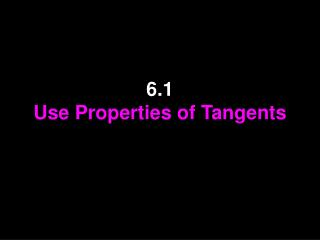Download Presentation6.1 Use Properties of Tangents# 6.1 Use Properties of Tangents - PowerPoint PPT Presentation

Download Presentation##### 6.1 Use Properties of Tangents

Download Policy: Content on the Website is provided to you AS IS for your information and personal use and may not be sold / licensed / shared on other websites without getting consent from its author. While downloading, if for some reason you are not able to download a presentation, the publisher may have deleted the file from their server.

- - - - - - - - - - - - - - - - - - - - - - - - - - - E N D - - - - - - - - - - - - - - - - - - - - - - - - - - -
##### Presentation Transcript

1. 6.1Use Properties of Tangents

2. Vocabulary • A circle is the set of all points in a plane that are equidistant from a given point called the center of the circle. • A radius is a segment whose endpoints are the center and any point on the circle.

3. Vocabulary • A chord is a segment whose endpoints are on a circle. • A diameter is a chord that contains the center of the circle. • The diameter is the longest chord a circle has.

4. Vocabulary • A secant is a line that intersects a circle in two points. • A tangent is a line in the plane of a circle that intersects the circle in exactly one point, the point of tangency.

5. Vocabulary • Theorem 6.1: • In a plane, a line is tangent to a circle if and only if the line is perpendicular to a radius of the circle at its endpoint on the circle.

6. Vocabulary • Theorem 6.2: • Tangent segments from a common external point are congruent.

7. Example 1: • Tell whether the line, ray, or segment is best described as a radius, chord, diameter, secant, or tangent of circle P.

8. Example 2: • Use the diagram to find the given lengths.

9. Example 3: • Tell how many common tangents the circles have and draw them.

10. Example 4: • RS is tangent to circle C at S and RT is tangent to circle C at T. • Find the value of x.

11. Example 5: • In the diagram, BC is a radius of circle C. Determine whether AB is tangent to circle C. Explain.

12. Example 6: • In the diagram, B is a point of tangency. Find the radius r of circle C. **Remember we know that the radius is perpendicular to the tangent** This makes a RIGHT triangle! So we can use Pythagorean theorem to solve for r.

13. Homework P. 186 #1, 9-11, 12-20even